Confidence Intervals

# 41 A Confidence Interval for A Population Proportion

During an election year, we see articles in the newspaper that state confidence intervals in terms of proportions or percentages. For example, a poll for a particular candidate running for president might show that the candidate has 40% of the vote within three percentage points (if the sample is large enough). Often, election polls are calculated with 95% confidence, so, the pollsters would be 95% confident that the true proportion of voters who favored the candidate would be between 0.37 and 0.43.

Investors in the stock market are interested in the true proportion of stocks that go up and down each week. Businesses that sell personal computers are interested in the proportion of households in the United States that own personal computers. Confidence intervals can be calculated for the true proportion of stocks that go up or down each week and for the true proportion of households in the United States that own personal computers.

The procedure to find the confidence interval for a population proportion is similar to that for the population mean, but the formulas are a bit different although conceptually identical. While the formulas are different, they are based upon the same mathematical foundation given to us by the Central Limit Theorem. Because of this we will see the same basic format using the same three pieces of information: the sample value of the parameter in question, the standard deviation of the relevant sampling distribution, and the number of standard deviations we need to have the confidence in our estimate that we desire.

How do you know you are dealing with a proportion problem? First, the underlying distribution has a binary random variable and therefore is a binomial distribution. (There is no mention of a mean or average.) If X is a binomial random variable, then X ~ B(n, p) where n is the number of trials and p is the probability of a success. To form a sample proportion, take X, the random variable for the number of successes and divide it by n, the number of trials (or the sample size). The random variable P′ (read “P prime”) is the sample proportion,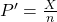(Sometimes the random variable is denoted as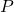, read “P hat”.)

p′ = the estimated proportion of successes or sample proportion of successes (p′ is a point estimate for p, the true population proportion, and thus q is the probability of a failure in any one trial.)

x = the number of successes in the sample

n = the size of the sample

The formula for the confidence interval for a population proportion follows the same format as that for an estimate of a population mean. Remembering the sampling distribution for the proportion from Chapter 7, the standard deviation was found to be: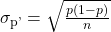The confidence interval for a population proportion, therefore, becomes: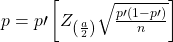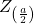is set according to our desired degree of confidence and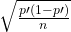is the standard deviation of the sampling distribution.

The sample proportions p′ and q′ are estimates of the unknown population proportions p and q. The estimated proportions p′ and q′ are used because p and q are not known.

Remember that as p moves further from 0.5 the binomial distribution becomes less symmetrical. Because we are estimating the binomial with the symmetrical normal distribution the further away from symmetrical the binomial becomes the less confidence we have in the estimate.

This conclusion can be demonstrated through the following analysis. Proportions are based upon the binomial probability distribution. The possible outcomes are binary, either “success” or “failure”. This gives rise to a proportion, meaning the percentage of the outcomes that are “successes”. It was shown that the binomial distribution could be fully understood if we knew only the probability of a success in any one trial, called p. The mean and the standard deviation of the binomial were found to be: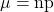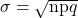It was also shown that the binomial could be estimated by the normal distribution if BOTH np AND nq were greater than 5. From the discussion above, it was found that the standardizing formula for the binomial distribution is: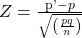which is nothing more than a restatement of the general standardizing formula with appropriate substitutions for μ and σ from the binomial. We can use the standard normal distribution, the reason Z is in the equation, because the normal distribution is the limiting distribution of the binomial. This is another example of the Central Limit Theorem. We have already seen that the sampling distribution of means is normally distributed. Recall the extended discussion in Chapter 7 concerning the sampling distribution of proportions and the conclusions of the Central Limit Theorem.

We can now manipulate this formula in just the same way we did for finding the confidence intervals for a mean, but to find the confidence interval for the binomial population parameter, p.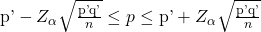Where p′ = x/n, the point estimate of p taken from the sample. Notice that p′ has replaced p in the formula. This is because we do not know p, indeed, this is just what we are trying to estimate.

Unfortunately, there is no correction factor for cases where the sample size is small so np′ and nq’ must always be greater than 5 to develop an interval estimate for p.

Suppose that a market research firm is hired to estimate the percent of adults living in a large city who have cell phones. Five hundred randomly selected adult residents in this city are surveyed to determine whether they have cell phones. Of the 500 people sampled, 421 responded yes – they own cell phones. Using a 95% confidence level, compute a confidence interval estimate for the true proportion of adult residents of this city who have cell phones.

• The solution step-by-step.

Let X = the number of people in the sample who have cell phones. X is binomial: the random variable is binary, people either have a cell phone or they do not.

To calculate the confidence interval, we must find p′, q′.

n = 500

x = the number of successes in the sample = 421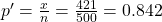p′ = 0.842 is the sample proportion; this is the point estimate of the population proportion.

q′ = 1 – p′ = 1 – 0.842 = 0.158

Since the requested confidence level is CL = 0.95, then α = 1 – CL = 1 – 0.95 = 0.05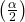= 0.025.

Then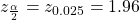This can be found using the Standard Normal probability table in (Figure). This can also be found in the students t table at the 0.025 column and infinity degrees of freedom because at infinite degrees of freedom the students t distribution becomes the standard normal distribution, Z.

The confidence interval for the true binomial population proportion is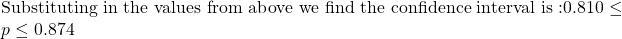InterpretationWe estimate with 95% confidence that between 81% and 87.4% of all adult residents of this city have cell phones.

Explanation of 95% Confidence LevelNinety-five percent of the confidence intervals constructed in this way would contain the true value for the population proportion of all adult residents of this city who have cell phones.

Try It

Suppose 250 randomly selected people are surveyed to determine if they own a tablet. Of the 250 surveyed, 98 reported owning a tablet. Using a 95% confidence level, compute a confidence interval estimate for the true proportion of people who own tablets.

(0.3315, 0.4525)

The Dundee Dog Training School has a larger than average proportion of clients who compete in competitive professional events. A confidence interval for the population proportion of dogs that compete in professional events from 150 different training schools is constructed. The lower limit is determined to be 0.08 and the upper limit is determined to be 0.16. Determine the level of confidence used to construct the interval of the population proportion of dogs that compete in professional events.

We begin with the formula for a confidence interval for a proportion because the random variable is binary; either the client competes in professional competitive dog events or they don’t.Next we find the sample proportion: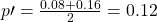The ± that makes up the confidence interval is thus 0.04; 0.12 + 0.04 = 0.16 and 0.12 − 0.04 = 0.08, the boundaries of the confidence interval. Finally, we solve for Z.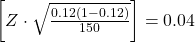, therefore Z = 1.51

And then look up the probability for 1.51 standard deviations on the standard normal table.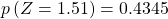,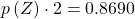or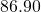.

A financial officer for a company wants to estimate the percent of accounts receivable that are more than 30 days overdue. He surveys 500 accounts and finds that 300 are more than 30 days overdue. Compute a 90% confidence interval for the true percent of accounts receivable that are more than 30 days overdue, and interpret the confidence interval.

• The solution is step-by-step:

x = 300 and n = 500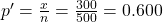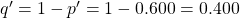Since confidence level = 0.90, then α = 1 – confidence level = (1 – 0.90) = 0.10= 0.05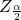= Z0.05 = 1.645

This Z-value can be found using a standard normal probability table. The student’s t-table can also be used by entering the table at the 0.05 column and reading at the line for infinite degrees of freedom. The t-distribution is the normal distribution at infinite degrees of freedom. This is a handy trick to remember in finding Z-values for commonly used levels of confidence. We use this formula for a confidence interval for a proportion:Substituting in the values from above we find the confidence interval for the true binomial population proportion is 0.564 ≤ p ≤ 0.636

Interpretation
• We estimate with 90% confidence that the true percent of all accounts receivable overdue 30 days is between 56.4% and 63.6%.
• Alternate Wording: We estimate with 90% confidence that between 56.4% and 63.6% of ALL accounts are overdue 30 days.

Explanation of 90% Confidence LevelNinety percent of all confidence intervals constructed in this way contain the true value for the population percent of accounts receivable that are overdue 30 days.

Try It

A student polls his school to see if students in the school district are for or against the new legislation regarding school uniforms. She surveys 600 students and finds that 480 are against the new legislation.

a. Compute a 90% confidence interval for the true percent of students who are against the new legislation, and interpret the confidence interval.

(0.7731, 0.8269); We estimate with 90% confidence that the true percent of all students in the district who are against the new legislation is between 77.31% and 82.69%.

b. In a sample of 300 students, 68% said they own an iPod and a smart phone. Compute a 97% confidence interval for the true percent of students who own an iPod and a smartphone.

Solution

Sixty-eight percent (68%) of students own an iPod and a smart phone.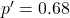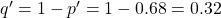Since CL = 0.97, we know α = 1 – 0.97 = 0.03 and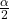= 0.015.

The area to the left of z0.015 is 0.015, and the area to the right of z0.015 is 1 – 0.015 = 0.985.

Using the TI 83, 83+, or 84+ calculator function InvNorm(.985,0,1),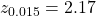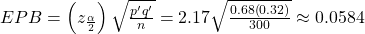p′ – EPB = 0.68 – 0.0584 = 0.0584

p′ + EPB = 0.68 + 0.0584 = 0.0584

We are 97% confident that the true proportion of all students who own an iPod and a smart phone is between 0.6216 and 0.7384.

### References

Jensen, Tom. “Democrats, Republicans Divided on Opinion of Music Icons.” Public Policy Polling. Available online at http://www.publicpolicypolling.com/Day2MusicPoll.pdf (accessed July 2, 2013).

Madden, Mary, Amanda Lenhart, Sandra Coresi, Urs Gasser, Maeve Duggan, Aaron Smith, and Meredith Beaton. “Teens, Social Media, and Privacy.” PewInternet, 2013. Available online at http://www.pewinternet.org/Reports/2013/Teens-Social-Media-And-Privacy.aspx (accessed July 2, 2013).

Prince Survey Research Associates International. “2013 Teen and Privacy Management Survey.” Pew Research Center: Internet and American Life Project. Available online at http://www.pewinternet.org/~/media//Files/Questionnaire/2013/Methods%20and%20Questions_Teens%20and%20Social%20Media.pdf (accessed July 2, 2013).

Saad, Lydia. “Three in Four U.S. Workers Plan to Work Pas Retirement Age: Slightly more say they will do this by choice rather than necessity.” Gallup® Economy, 2013. Available online at http://www.gallup.com/poll/162758/three-four-workers-plan-work-past-retirement-age.aspx (accessed July 2, 2013).

The Field Poll. Available online at http://field.com/fieldpollonline/subscribers/ (accessed July 2, 2013).

Zogby. “New SUNYIT/Zogby Analytics Poll: Few Americans Worry about Emergency Situations Occurring in Their Community; Only one in three have an Emergency Plan; 70% Support Infrastructure ‘Investment’ for National Security.” Zogby Analytics, 2013. Available online at http://www.zogbyanalytics.com/news/299-americans-neither-worried-nor-prepared-in-case-of-a-disaster-sunyit-zogby-analytics-poll (accessed July 2, 2013).

“52% Say Big-Time College Athletics Corrupt Education Process.” Rasmussen Reports, 2013. Available online at http://www.rasmussenreports.com/public_content/lifestyle/sports/may_2013/52_say_big_time_college_athletics_corrupt_education_process (accessed July 2, 2013).

### Chapter Review

Some statistical measures, like many survey questions, measure qualitative rather than quantitative data. In this case, the population parameter being estimated is a proportion. It is possible to create a confidence interval for the true population proportion following procedures similar to those used in creating confidence intervals for population means. The formulas are slightly different, but they follow the same reasoning.

Let p′ represent the sample proportion, x/n, where x represents the number of successes and n represents the sample size. Let q′ = 1 – p′. Then the confidence interval for a population proportion is given by the following formula:### Formula Review

p′=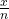where x represents the number of successes in a sample and n represents the sample size. The variable p′ is the sample proportion and serves as the point estimate for the true population proportion.

q′ = 1 – p

The variable p′ has a binomial distribution that can be approximated with the normal distribution shown here. The confidence interval for the true population proportion is given by the formula: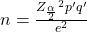provides the number of observations needed to sample to estimate the population proportion, p, with confidence 1 – α and margin of error e. Where e = the acceptable difference between the actual population proportion and the sample proportion.

Use the following information to answer the next two exercises: Marketing companies are interested in knowing the population percent of women who make the majority of household purchasing decisions.

When designing a study to determine this population proportion, what is the minimum number you would need to survey to be 90% confident that the population proportion is estimated to within 0.05?

If it were later determined that it was important to be more than 90% confident and a new survey were commissioned, how would it affect the minimum number you need to survey? Why?

It would decrease, because the z-score would decrease, which reducing the numerator and lowering the number.

Use the following information to answer the next five exercises: Suppose the marketing company did do a survey. They randomly surveyed 200 households and found that in 120 of them, the woman made the majority of the purchasing decisions. We are interested in the population proportion of households where women make the majority of the purchasing decisions.

Identify the following:

1. x = ______
2. n = ______
3. p′ = ______

Define the random variables X and P′ in words.

X is the number of “successes” where the woman makes the majority of the purchasing decisions for the household. P′ is the percentage of households sampled where the woman makes the majority of the purchasing decisions for the household.

Which distribution should you use for this problem?

Construct a 95% confidence interval for the population proportion of households where the women make the majority of the purchasing decisions. State the confidence interval, sketch the graph, and calculate the error bound.

CI: (0.5321, 0.6679)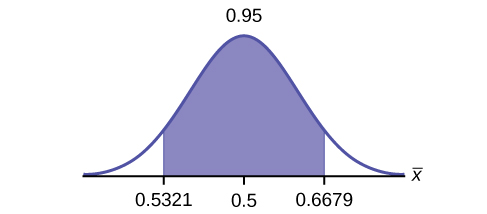EBM: 0.0679

List two difficulties the company might have in obtaining random results, if this survey were done by email.

Use the following information to answer the next five exercises: Of 1,050 randomly selected adults, 360 identified themselves as manual laborers, 280 identified themselves as non-manual wage earners, 250 identified themselves as mid-level managers, and 160 identified themselves as executives. In the survey, 82% of manual laborers preferred trucks, 62% of non-manual wage earners preferred trucks, 54% of mid-level managers preferred trucks, and 26% of executives preferred trucks.

We are interested in finding the 95% confidence interval for the percent of executives who prefer trucks. Define random variables X and P′ in words.

X is the number of “successes” where an executive prefers a truck. P′ is the percentage of executives sampled who prefer a truck.

Which distribution should you use for this problem?

Construct a 95% confidence interval. State the confidence interval, sketch the graph, and calculate the error bound.

CI: (0.19432, 0.33068)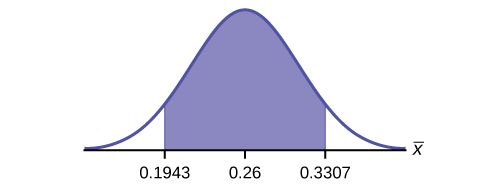Suppose we want to lower the sampling error. What is one way to accomplish that?

The sampling error given in the survey is ±2%. Explain what the ±2% means.

The sampling error means that the true mean can be 2% above or below the sample mean.

Use the following information to answer the next five exercises: A poll of 1,200 voters asked what the most significant issue was in the upcoming election. Sixty-five percent answered the economy. We are interested in the population proportion of voters who feel the economy is the most important.

Define the random variable X in words.

Define the random variable P′ in words.

P′ is the proportion of voters sampled who said the economy is the most important issue in the upcoming election.

Which distribution should you use for this problem?

Construct a 90% confidence interval, and state the confidence interval and the error bound.

CI: (0.62735, 0.67265)

EBM: 0.02265

What would happen to the confidence interval if the level of confidence were 95%?

Use the following information to answer the next 16 exercises: The Ice Chalet offers dozens of different beginning ice-skating classes. All of the class names are put into a bucket. The 5 P.M., Monday night, ages 8 to 12, beginning ice-skating class was picked. In that class were 64 girls and 16 boys. Suppose that we are interested in the true proportion of girls, ages 8 to 12, in all beginning ice-skating classes at the Ice Chalet. Assume that the children in the selected class are a random sample of the population.

What is being counted?

The number of girls, ages 8 to 12, in the 5 P.M. Monday night beginning ice-skating class.

In words, define the random variable X.

Calculate the following:

1. x = _______
2. n = _______
3. p′ = _______
1. x = 64
2. n = 80
3. p′ = 0.8

State the estimated distribution of X. X~________

Define a new random variable P′. What is p′ estimating?

p

In words, define the random variable P′.

State the estimated distribution of P′. Construct a 92% Confidence Interval for the true proportion of girls in the ages 8 to 12 beginning ice-skating classes at the Ice Chalet.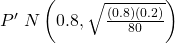. (0.72171, 0.87829).

How much area is in both tails (combined)?

How much area is in each tail?

0.04

Calculate the following:

1. lower limit
2. upper limit
3. error bound

The 92% confidence interval is _______.

(0.72; 0.88)

Fill in the blanks on the graph with the areas, upper and lower limits of the confidence interval, and the sample proportion.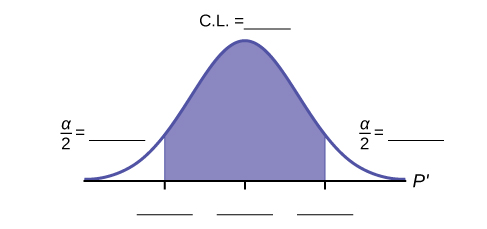In one complete sentence, explain what the interval means.

With 92% confidence, we estimate the proportion of girls, ages 8 to 12, in a beginning ice-skating class at the Ice Chalet to be between 72% and 88%.

Using the same p′ and level of confidence, suppose that n were increased to 100. Would the error bound become larger or smaller? How do you know?

Using the same p′ and n = 80, how would the error bound change if the confidence level were increased to 98%? Why?

The error bound would increase. Assuming all other variables are kept constant, as the confidence level increases, the area under the curve corresponding to the confidence level becomes larger, which creates a wider interval and thus a larger error.

If you decreased the allowable error bound, why would the minimum sample size increase (keeping the same level of confidence)?

### Homework

Insurance companies are interested in knowing the population percent of drivers who always buckle up before riding in a car.

1. When designing a study to determine this population proportion, what is the minimum number you would need to survey to be 95% confident that the population proportion is estimated to within 0.03?
2. If it were later determined that it was important to be more than 95% confident and a new survey was commissioned, how would that affect the minimum number you would need to survey? Why?
1. 1,068
2. The sample size would need to be increased since the critical value increases as the confidence level increases.

Suppose that the insurance companies did do a survey. They randomly surveyed 400 drivers and found that 320 claimed they always buckle up. We are interested in the population proportion of drivers who claim they always buckle up.

1. x = __________
2. n = __________
3. p′ = __________
1. Define the random variables X and P′, in words.
2. Which distribution should you use for this problem? Explain your choice.
3. Construct a 95% confidence interval for the population proportion who claim they always buckle up.
1. State the confidence interval.
2. Sketch the graph.
4. If this survey were done by telephone, list three difficulties the companies might have in obtaining random results.

According to a recent survey of 1,200 people, 61% feel that the president is doing an acceptable job. We are interested in the population proportion of people who feel the president is doing an acceptable job.

1. Define the random variables X and P′ in words.
2. Which distribution should you use for this problem? Explain your choice.
3. Construct a 90% confidence interval for the population proportion of people who feel the president is doing an acceptable job.
1. State the confidence interval.
2. Sketch the graph.
1. X = the number of people who feel that the president is doing an acceptable job;

P′ = the proportion of people in a sample who feel that the president is doing an acceptable job.

2.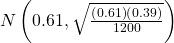1. CI: (0.59, 0.63)
2. Check student’s solution

An article regarding interracial dating and marriage recently appeared in the Washington Post. Of the 1,709 randomly selected adults, 315 identified themselves as Latinos, 323 identified themselves as blacks, 254 identified themselves as Asians, and 779 identified themselves as whites. In this survey, 86% of blacks said that they would welcome a white person into their families. Among Asians, 77% would welcome a white person into their families, 71% would welcome a Latino, and 66% would welcome a black person.

1. We are interested in finding the 95% confidence interval for the percent of all black adults who would welcome a white person into their families. Define the random variables X and P′, in words.
2. Which distribution should you use for this problem? Explain your choice.
3. Construct a 95% confidence interval.
1. State the confidence interval.
2. Sketch the graph.

Refer to the information in (Figure).

1. Construct three 95% confidence intervals.
1. percent of all Asians who would welcome a white person into their families.
2. percent of all Asians who would welcome a Latino into their families.
3. percent of all Asians who would welcome a black person into their families.
2. Even though the three point estimates are different, do any of the confidence intervals overlap? Which?
3. For any intervals that do overlap, in words, what does this imply about the significance of the differences in the true proportions?
4. For any intervals that do not overlap, in words, what does this imply about the significance of the differences in the true proportions?
1. (0.72, 0.82)
2. (0.65, 0.76)
3. (0.60, 0.72)
1. Yes, the intervals (0.72, 0.82) and (0.65, 0.76) overlap, and the intervals (0.65, 0.76) and (0.60, 0.72) overlap.
2. We can say that there does not appear to be a significant difference between the proportion of Asian adults who say that their families would welcome a white person into their families and the proportion of Asian adults who say that their families would welcome a Latino person into their families.
3. We can say that there is a significant difference between the proportion of Asian adults who say that their families would welcome a white person into their families and the proportion of Asian adults who say that their families would welcome a black person into their families.

Stanford University conducted a study of whether running is healthy for men and women over age 50. During the first eight years of the study, 1.5% of the 451 members of the 50-Plus Fitness Association died. We are interested in the proportion of people over 50 who ran and died in the same eight-year period.

1. Define the random variables X and P′ in words.
2. Which distribution should you use for this problem? Explain your choice.
3. Construct a 97% confidence interval for the population proportion of people over 50 who ran and died in the same eight–year period.
1. State the confidence interval.
2. Sketch the graph.
4. Explain what a “97% confidence interval” means for this study.

A telephone poll of 1,000 adult Americans was reported in an issue of Time Magazine. One of the questions asked was “What is the main problem facing the country?” Twenty percent answered “crime.” We are interested in the population proportion of adult Americans who feel that crime is the main problem.

1. Define the random variables X and P′ in words.
2. Which distribution should you use for this problem? Explain your choice.
3. Construct a 95% confidence interval for the population proportion of adult Americans who feel that crime is the main problem.
1. State the confidence interval.
2. Sketch the graph.
4. Suppose we want to lower the sampling error. What is one way to accomplish that?
5. The sampling error given by Yankelovich Partners, Inc. (which conducted the poll) is ±3%. In one to three complete sentences, explain what the ±3% represents.
1. X = the number of adult Americans who feel that crime is the main problem; P′ = the proportion of adult Americans who feel that crime is the main problem
2. Since we are estimating a proportion, given P′ = 0.2 and n = 1000, the distribution we should use is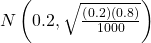.
1. CI: (0.18, 0.22)
2. Check student’s solution.
3. One way to lower the sampling error is to increase the sample size.
4. The stated “± 3%” represents the maximum error bound. This means that those doing the study are reporting a maximum error of 3%. Thus, they estimate the percentage of adult Americans who feel that crime is the main problem to be between 18% and 22%.

Refer to (Figure). Another question in the poll was “[How much are] you worried about the quality of education in our schools?” Sixty-three percent responded “a lot”. We are interested in the population proportion of adult Americans who are worried a lot about the quality of education in our schools.

1. Define the random variables X and P′ in words.
2. Which distribution should you use for this problem? Explain your choice.
3. Construct a 95% confidence interval for the population proportion of adult Americans who are worried a lot about the quality of education in our schools.
1. State the confidence interval.
2. Sketch the graph.
4. The sampling error given by Yankelovich Partners, Inc. (which conducted the poll) is ±3%. In one to three complete sentences, explain what the ±3% represents.

Use the following information to answer the next three exercises: According to a Field Poll, 79% of California adults (actual results are 400 out of 506 surveyed) feel that “education and our schools” is one of the top issues facing California. We wish to construct a 90% confidence interval for the true proportion of California adults who feel that education and the schools is one of the top issues facing California.

A point estimate for the true population proportion is:

1. 0.90
2. 1.27
3. 0.79
4. 400

c

A 90% confidence interval for the population proportion is _______.

1. (0.761, 0.820)
2. (0.125, 0.188)
3. (0.755, 0.826)
4. (0.130, 0.183)

Use the following information to answer the next two exercises: Five hundred and eleven (511) homes in a certain southern California community are randomly surveyed to determine if they meet minimal earthquake preparedness recommendations. One hundred seventy-three (173) of the homes surveyed met the minimum recommendations for earthquake preparedness, and 338 did not.

Find the confidence interval at the 90% Confidence Level for the true population proportion of southern California community homes meeting at least the minimum recommendations for earthquake preparedness.

1. (0.2975, 0.3796)
2. (0.6270, 0.6959)
3. (0.3041, 0.3730)
4. (0.6204, 0.7025)

The point estimate for the population proportion of homes that do not meet the minimum recommendations for earthquake preparedness is ______.

1. 0.6614
2. 0.3386
3. 173
4. 338

a

On May 23, 2013, Gallup reported that of the 1,005 people surveyed, 76% of U.S. workers believe that they will continue working past retirement age. The confidence level for this study was reported at 95% with a ±3% margin of error.

1. Determine the estimated proportion from the sample.
2. Determine the sample size.
3. Identify CL and α.
4. Calculate the error bound based on the information provided.
5. Compare the error bound in part d to the margin of error reported by Gallup. Explain any differences between the values.
6. Create a confidence interval for the results of this study.
7. A reporter is covering the release of this study for a local news station. How should she explain the confidence interval to her audience?

A national survey of 1,000 adults was conducted on May 13, 2013 by Rasmussen Reports. It concluded with 95% confidence that 49% to 55% of Americans believe that big-time college sports programs corrupt the process of higher education.

1. Find the point estimate and the error bound for this confidence interval.
2. Can we (with 95% confidence) conclude that more than half of all American adults believe this?
3. Use the point estimate from part a and n = 1,000 to calculate a 75% confidence interval for the proportion of American adults that believe that major college sports programs corrupt higher education.
4. Can we (with 75% confidence) conclude that at least half of all American adults believe this?
1. p′ =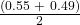= 0.52; EBP = 0.55 – 0.52 = 0.03
2. No, the confidence interval includes values less than or equal to 0.50. It is possible that less than half of the population believe this.
3. CL = 0.75, so α = 1 – 0.75 = 0.25 and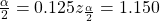. (The area to the right of this z is 0.125, so the area to the left is 1 – 0.125 = 0.875.)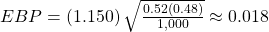(p′ – EBP, p′ + EBP) = (0.52 – 0.018, 0.52 + 0.018) = (0.502, 0.538)
4. Yes – this interval does not fall less than 0.50 so we can conclude that at least half of all American adults believe that major sports programs corrupt education – but we do so with only 75% confidence.

1. Create a 99% confidence interval for the true proportion of American adults who have illegally downloaded music.
2. This survey was conducted through automated telephone interviews on May 6 and 7, 2013. The error bound of the survey compensates for sampling error, or natural variability among samples. List some factors that could affect the survey’s outcome that are not covered by the margin of error.
3. Without performing any calculations, describe how the confidence interval would change if the confidence level changed from 99% to 90%.

You plan to conduct a survey on your college campus to learn about the political awareness of students. You want to estimate the true proportion of college students on your campus who voted in the 2012 presidential election with 95% confidence and a margin of error no greater than five percent. How many students must you interview?

### Key Terms

Binomial Distribution
a discrete random variable (RV) which arises from Bernoulli trials; there are a fixed number, n, of independent trials. “Independent” means that the result of any trial (for example, trial 1) does not affect the results of the following trials, and all trials are conducted under the same conditions. Under these circumstances the binomial RV X is defined as the number of successes in n trials. The notation is: X~B(n,p). The mean is μ = np and the standard deviation is σ =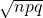. The probability of exactly x successes in n trials is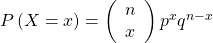.
Error Bound for a Population Proportion (EBP)
the margin of error; depends on the confidence level, the sample size, and the estimated (from the sample) proportion of successes.